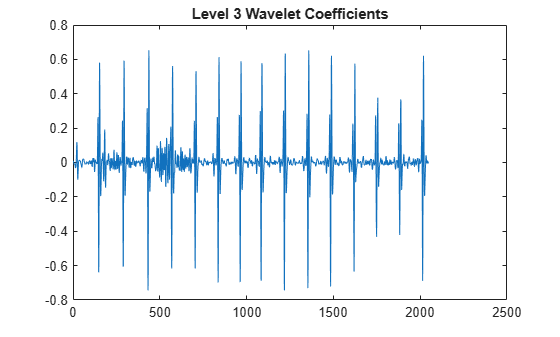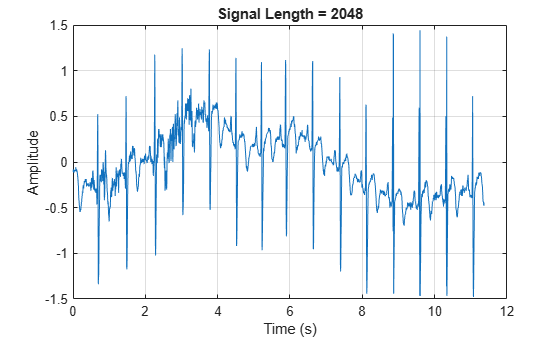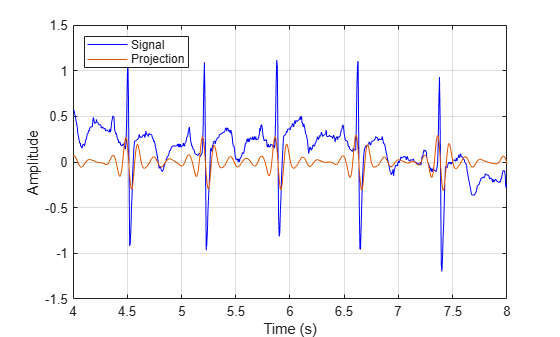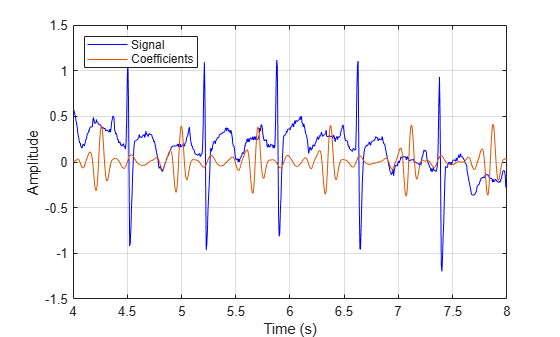Documentation

# modwt

Maximal overlap discrete wavelet transform

## Syntax

``w = modwt(x)``
``w = modwt(x,wname)``
``w = modwt(x,Lo,Hi)``
``w = modwt(___,lev)``
``w = modwt(___,'reflection')``

## Description

example

````w = modwt(x)` returns the maximal overlap discrete wavelet transform (MODWT) of the 1-D real-valued signal, `x`. ```

example

````w = modwt(x,wname)` uses the orthogonal wavelet, `wname`, for the MODWT. ```

example

````w = modwt(x,Lo,Hi)` uses the scaling filter, `Lo`, and wavelet filter, `Hi`, to compute the MODWT. These filters must satisfy the conditions for an orthogonal wavelet. You cannot specify `Lo` and `Hi` if you specify `wname`.```

example

``` `w = modwt(___,lev)` computes the MODWT down to the specified level, `lev`, using any of the arguments from previous syntaxes. ```

example

````w = modwt(___,'reflection')` computes the MODWT using reflection boundary handling. Other inputs can be any of the arguments from previous syntaxes. Before computing the wavelet transform, `modwt` extends the signal symmetrically at the right boundary to twice the signal length, `[x flip(x)]`. The number of wavelet and scaling coefficients that `modwt` returns is equal to twice the length of the input signal. By default, the signal is extended periodically.```

## Examples

collapse all

Obtain the MODWT of an electrocardiogram (ECG) signal using the default `sym4` wavelet down to the maximum level.

```load wecg; wtecg = modwt(wecg);```

wtecg is 12-by-2048 matrix. The first eleven rows are the wavelet coefficients for scales ${2}^{1}$ to ${2}^{11}$. The final row contains the scaling coefficients at scale ${2}^{11}$. Plot the detail (wavelet) coefficients for scale ${2}^{3}$.

```plot(wtecg(3,:)) title('Level 3 Wavelet Coefficients')```Obtain the MODWT of Southern Oscillation Index data with the `'db2'` wavelet down to the maximum level.

```load soi; wsoi = modwt(soi,'db2');```

Obtain the MODWT of the Deutsche Mark - U.S. Dollar exchange rate data using the Fejer-Korovkin length 8 scaling and wavelet filters.

```load DM_USD; [Lo,Hi] = wfilters('fk8'); wdm = modwt(DM_USD,Lo,Hi);```

Obtain the MODWT of an ECG signal down to scale ${2}^{4}$, which corresponds to level four. Use the default `'sym4'` wavelet.

```load wecg; wtecg = modwt(wecg,4);```

`wtecg` is a 5-by-2048 matrix. The row size is L+1, where, in this case, the level (L) is 4. The column size matched the number of input samples.

Obtain the MODWT of an ECG signal using reflection boundary handling. Use the default `'sym4'` wavelet and obtain the transform down to level 4.

```load wecg; wtecg = modwt(wecg,4,'reflection');```

`wtecg` has 4096 columns, which is twice the length of the input signal, `wecg`.

This example demonstrates the differences between the functions MODWT and MODWTMRA. The MODWT partitions a signal's energy across detail coefficients and scaling coefficients. The MODWTMRA projects a signal onto wavelet subspaces and a scaling subspace.

Choose the 'sym6' wavelet. Load and plot an ECG waveform. The ECG data is taken from the MIT-BIH Arrhythmia Database.

```load mit200 wv = 'sym6'; plot(ecgsig) grid on title(['Signal Length = ',num2str(length(ecgsig))])```Take the MODWT of the signal.

`wtecg = modwt(ecgsig,wv);`

The input data are samples of a function $f\left(x\right)$ evaluated at $N$-many time points. The function can be expressed as a linear combination of the scaling function $\varphi \left(x\right)$ and wavelet $\psi \left(x\right)$at varying scales and translations: $f\left(x\right)=\sum _{k=0}^{N-1}{c}_{k}\phantom{\rule{0.16666666666666666em}{0ex}}{2}^{-{J}_{0}/2}\varphi \left({2}^{-{J}_{0}}\phantom{\rule{0.16666666666666666em}{0ex}}x-k\right)+\sum _{j=1}^{{J}_{0}}{f}_{j}\left(x\right)$ where ${f}_{j}\left(x\right)=\sum _{k=0}^{N-1}{d}_{j,k}\phantom{\rule{0.16666666666666666em}{0ex}}{2}^{-j/2}\phantom{\rule{0.16666666666666666em}{0ex}}\psi \left({2}^{-j}x-k\right)$ and ${J}_{0}$ is the number of levels of wavelet decomposition. The first sum is the coarse scale approximation of the signal, and the ${f}_{j}\left(x\right)$ are the details at successive scales. MODWT returns the $N$-many coefficients $\left\{{c}_{k}\right\}$and the $\left({J}_{0}×N\right)$-many detail coefficients $\left\{{d}_{j,k}\right\}$ of the expansion. Each row in `wtecg` contains the coefficients at a different scale.

When taking the MODWT of a signal of length $N$, there are $\text{floor}\left({\mathrm{log}}_{2}\left(N\right)\right)$-many levels of decomposition (by default). Detail coefficients are produced at each level. Scaling coefficients are returned only for the final level. In this example, since $N=10000$, ${J}_{0}=\text{floor}\left(\mathrm{log}2\left(10000\right)\right)=13$ and the number of rows in `wtecg` is ${J}_{0}+1=13+1=14$.

The MODWT partitions the energy across the various scales and scaling coefficients: ${||X||}^{2}=\sum _{j=1}^{{J}_{0}}{||{W}_{j}||}^{2}+{||{V}_{{J}_{0}}||}^{2}$ where $X$ is the input data, ${W}_{j}$ are the detail coefficients at scale $j$, and ${V}_{{J}_{0}}$ are the final-level scaling coefficients.

Compute the energy at each scale, and evaluate their sum.

```energy_by_scales = sum(wtecg.^2,2); Levels = {'D1';'D2';'D3';'D4';'D5';'D6';'D7';'D8';'D9';'D10';'D11';'D12';'D13';'A13'}; energy_table = table(Levels,energy_by_scales); disp(energy_table)```
``` Levels energy_by_scales _______ ________________ {'D1' } 0.31592 {'D2' } 2.6504 {'D3' } 28.802 {'D4' } 159.37 {'D5' } 300.5 {'D6' } 431.33 {'D7' } 444.93 {'D8' } 182.37 {'D9' } 45.381 {'D10'} 11.578 {'D11'} 19.809 {'D12'} 4.5406 {'D13'} 3.308 {'A13'} 192.46 ```
`energy_total = varfun(@sum,energy_table(:,2))`
```energy_total=1×1 table sum_energy_by_scales ____________________ 1827.3 ```

Confirm the MODWT is energy-preserving by computing the energy of the signal and comparing it with the sum of the energies over all scales.

```energy_ecg = sum(ecgsig.^2); max(abs(energy_total.sum_energy_by_scales-energy_ecg))```
```ans = 4.0875e-09 ```

Take the MODWTMRA of the signal.

`mraecg = modwtmra(wtecg,wv);`

MODWTMRA returns the projections of the function $f\left(x\right)$ onto the various wavelet subspaces and final scaling space. That is, MODWTMRA returns $\sum _{k=0}^{N-1}{c}_{k}\phantom{\rule{0.16666666666666666em}{0ex}}{2}^{-{J}_{0}/2}\varphi \left({2}^{-{J}_{0}}\phantom{\rule{0.16666666666666666em}{0ex}}x-k\right)$and the ${J}_{0}$-many $\left\{{f}_{j}\left(x\right)\right\}$evaluated at $N$-many time points. Each row in `mraecg` is a projection of $f\left(x\right)$ onto a different subspace. This means the original signal can be recovered by adding all the projections. This is not true in the case of the MODWT. Adding the coefficients in `wtecg` will not recover the original signal.

Choose a time point, add the projections of $f\left(x\right)$ evaluated at that time point and compare with the original signal.

```time_point = 1000; abs(sum(mraecg(:,time_point))-ecgsig(time_point))```
```ans = 3.0942e-13 ```

Confirm that, unlike MODWT, MODWTMRA is not an energy-preserving transform.

```energy_ecg = sum(ecgsig.^2); energy_mra_scales = sum(mraecg.^2,2); energy_mra = sum(energy_mra_scales); max(abs(energy_mra-energy_ecg))```
```ans = 534.7949 ```

The MODWTMRA is a zero-phase filtering of the signal. Features will be time-aligned. Demonstrate this by plotting the original signal and one of its projections. To better illustrate the alignment, zoom in.

```figure plot(ecgsig) hold on plot(mraecg(4,:),'-') grid on xlim([4000 5000]) legend('Signal','Projection','Location','northwest')```Make a similar plot using the MODWT coefficients at the same scale. Note that features will not be time-aligned. The MODWT is not a zero-phase filtering of the input.

```figure plot(ecgsig) hold on plot(wtecg(4,:),'-') grid on xlim([4000 5000]) legend('Signal','Coefficients','Location','northwest')```References

Goldberger A. L., L. A. N. Amaral, L. Glass, J. M. Hausdorff, P. Ch. Ivanov, R. G. Mark, J. E. Mietus, G. B. Moody, C-K Peng, H. E. Stanley. "PhysioBank, PhysioToolkit, and PhysioNet: Components of a New Research Resource for Complex Physiologic Signals." Circulation 101. Vol.23, e215-e220, 2000. `http://circ.ahajournals.org/cgi/content/full/101/23/e215`

Moody, G. B. "Evaluating ECG Analyzers". `http://www.physionet.org/physiotools/wfdb/doc/wag-src/eval0.tex`

Moody G. B., R. G. Mark. "The impact of the MIT-BIH Arrhythmia Database." IEEE Eng in Med and Biol. Vol. 20, Number 3, 2001), pp. 45-50 .

## Input Arguments

collapse all

Input signal, specified as a row or column vector. `x` must have at least two elements.

By default, `modwt` computes the wavelet transform down to level `floor(log2(length(x)))` using the Daubechies least-asymmetric wavelet with four vanishing moments (`'sym4'`) and periodic boundary handling.

Data Types: `double`

Analyzing wavelet, specified as one of the following:

• `'haar'` — Haar wavelet

• `'dbN'` — Extremal phase Daubechies wavelet with `N` vanishing moments, where `N` is a positive integer from 1 to 45.

• `'symN'` — Symlets wavelet with `N` vanishing moments, where `N` is a positive integer from 2 to 45.

• `'coifN'` — Coiflets wavelet with `N` vanishing moments, where `N` is a positive integer from 1 to 5.

• `'fkN'` — Fejér-Korovkin wavelet with `N` coefficients, where `N = 4, 6, 8, 14, 18` and `22`.

Scaling filter, specified as an even-length real-valued vector. `Lo` must satisfy the conditions necessary to generate an orthogonal scaling function. You can specify `Lo` only if you do not specify `wname`.

Wavelet filter, specified as an even-length real-valued vector. `Hi` must satisfy the conditions necessary to generate an orthogonal wavelet. You can specify `Hi` only if you do not specify `wname`.

Transform level, specified as a positive integer less than or equal to `floor(log2(length(x)))`.

## Output Arguments

collapse all

Wavelet transform , returned as an L+1-by-N matrix containing wavelet coefficients and final-level scaling coefficients. L is the level of the MODWT. N is equal to the input signal length unless you specify `'reflection'` boundary handling, in which case N is twice the length of the input signal. The kth row of `w` contains the wavelet coefficients for scale 2k (wavelet scale 2(k-1)). The final, (L+1)th, row of `w` contains the scaling coefficients for scale 2L.

## Algorithms

The standard algorithm for the MODWT implements the circular convolution directly in the time domain. This implementation of the MODWT performs the circular convolution in the Fourier domain. The wavelet and scaling filter coefficients at level j are computed by taking the inverse discrete Fourier transform (DFT) of a product of DFTs. The DFTs in the product are the signal’s DFT and the DFT of the jth level wavelet or scaling filter.

Let Hk and Gk denote the length N DFTs of the MODWT wavelet and scaling filters, respectively. Let j denote the level and N denote the sample size.

The jth level wavelet filter is defined by

`$\frac{1}{N}\sum _{k=0}^{N-1}{H}_{j,k}{e}^{i2\pi nk/N}$`

where

`${H}_{j,k}={H}_{{2}^{j-1}k\text{mod}N}\prod _{m=0}^{j-2}{G}_{{2}^{m}k\text{mod}N}$`

The jth level scaling filter is

`$\frac{1}{N}\sum _{k=0}^{N-1}{G}_{j,k}{e}^{i2\pi nk/N}$`

where

`${G}_{j,k}=\prod _{m=0}^{j-1}{G}_{{2}^{m}k\text{mod}N}$`

 Percival, D. B., and A. T. Walden. Wavelet Methods for Time Series Analysis. Cambridge, UK: Cambridge University Press, 2000.

 Percival, D. B., and H. O. Mofjeld. “Analysis of subtidal coastal sea level fluctuations using wavelets.”Journal of the American Statistical Association. Vol. 92, pp 868–880.

##### Support평가판 신청